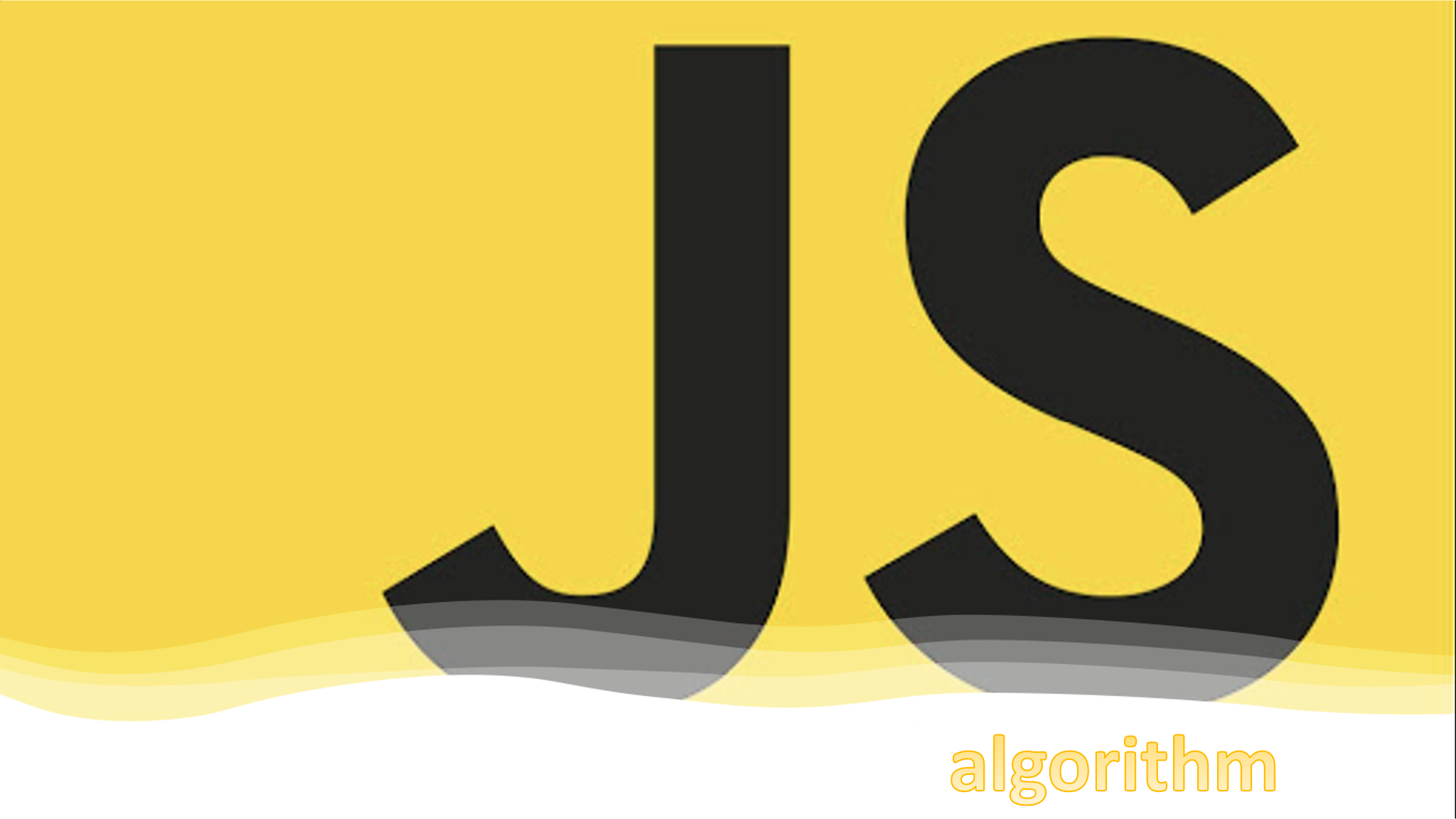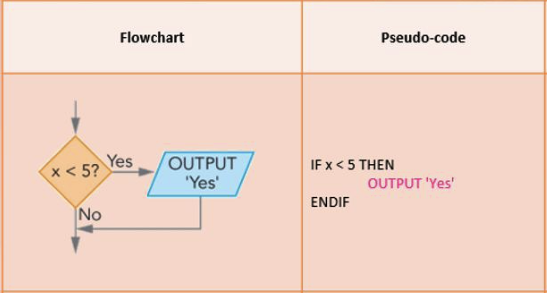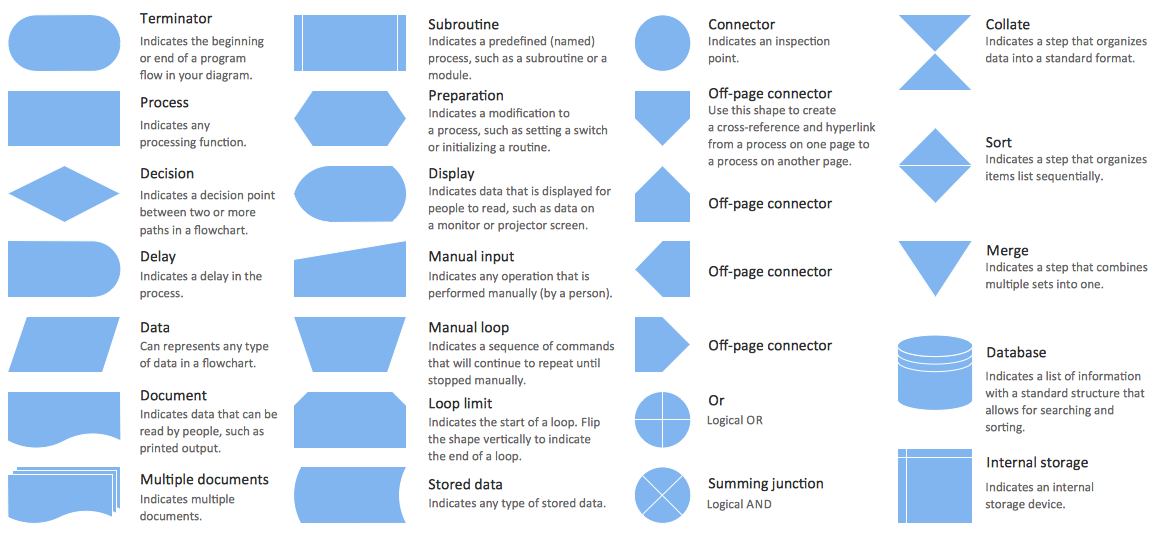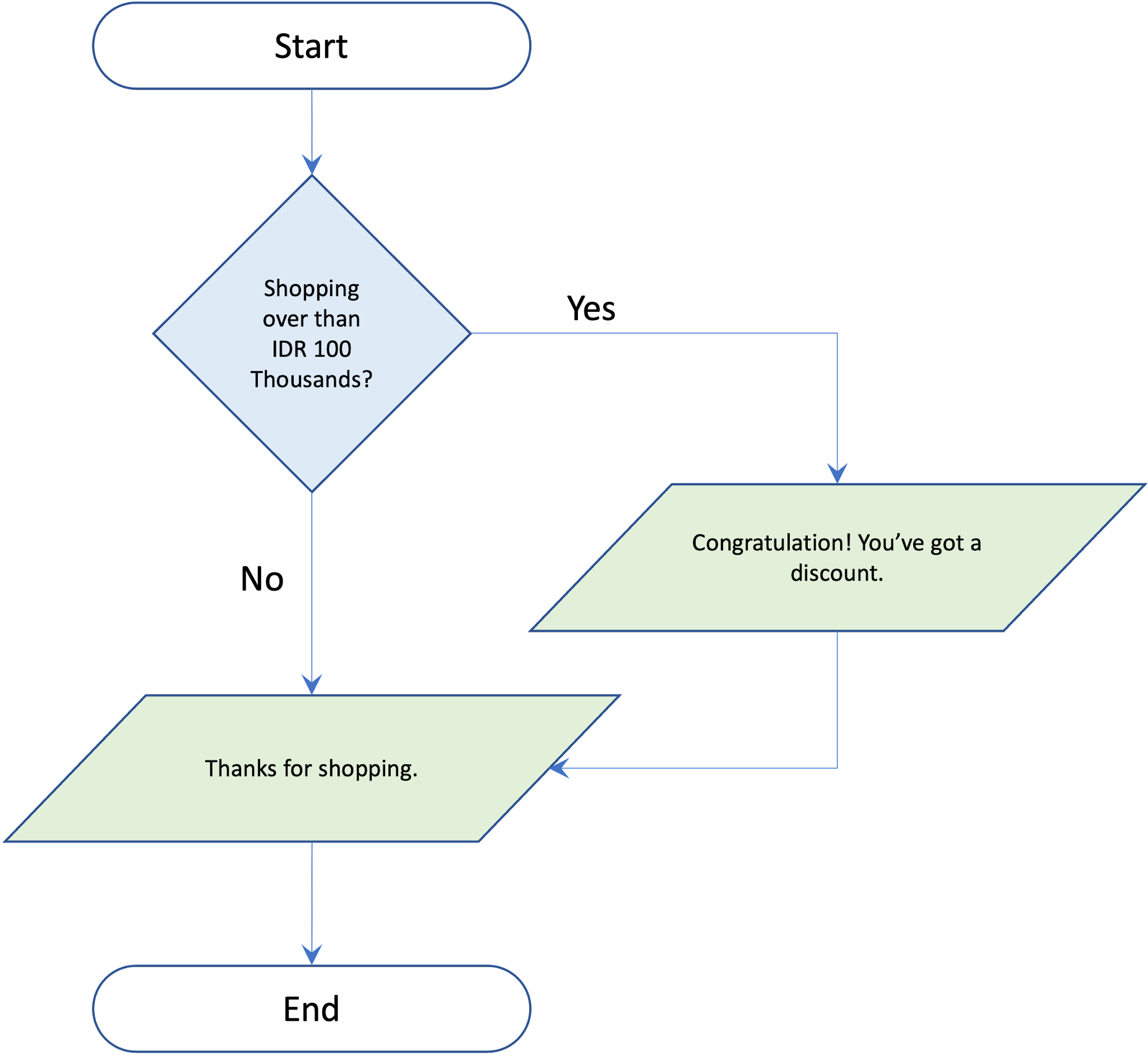### JavaScript AlgorithmAlgorithm is a step by step method to solve a problem. When we think of solving a problem step by step, we are actually implementing an algorithm. Before writing code, we should first determine how to make the algorithm flow. There are 2 options in compiling the algorithm flow, by Flowchart or Pseudo-code.Algorithm Writing Methods

Flowchart
Flowchart is a visual representation of the control flow of an algorithm. This representation defines several things, such as: statements that need to be executed, decisions that need to be made, flow logic (for iteration and other purposes), and terminal that shows the starting and ending points.Flowchart Symbols

And below is a sample of flowchart.Sample of Flowchart

Pros (+)
1. Flowchart is able to present control flow algorithm visually. So, it is easier for us to understand the flow of solving a problem or condition.
2. It is easier to detect errors or inaccuracies in a complex and detailed flowchart.

Cons (-)
1. The work is time consuming. We have to position, label, and connect the symbols in the flowchart.
2. If you use special tools to create flowcharts, it will hinder our understanding of algorithms.

Pseudo-code
Pseudo-code is text or words to indicate a flow.
Eg.
READ "the first number" and SAVE in the variable (A)
READ "the second number" and SAVE in the variable (B)
SUM the both numbers
SAVE the result in the variable (SUM)
PRINT the result (SUM)

After generating the pseudocode, how do you create a decision flow?

1. if-else
The if(...) statement evaluates the condition in parentheses (). If the result is true, then the code contained in the block will be executed.
Eg.
let year = prompt('What year the independence of Indonesia?');
if (year == 1945) {
} else {
}

2. Operator Conditional '?'
Sometimes we need to assign a dependent variable to a condition.
Eg.
let result;
let age = prompt('How old are you?');
if (age > 18) {
result = true;
} else {
result = false;
}

3.  switch-case
In the previous discussion, we have learned about using if-else. However, the use of if-else can be changed to a simpler form if a condition has many choices. We can do this with the statement switch-case.
Eg.
let a = 2 + 2;
switch (a) {
case 3:
break;
case 4:
break;
case 5:
break;
default:
}

4. Looping flow
Every programming allows us to repeat an action. By implementing loops, it becomes easier for us to use the same code in multiple times. There are three types of looping flow that we need to know, such as: for, while and do...while

a. 'for' syntax
Concept:
for (initial; condition; value changer) {
... statement ...
}
Eg.
for (let i = 1; i <= 5; i++) {
}

b. 'while' syntax
Concept:
while (condition) {
... statement ...
}
Eg.
let i = 1;
while (i<=5) {
i++;
}

c. 'do...while' syntax
Concept:
do {
... statement ...
} while (condition);
Eg.
let i = 1;
do {